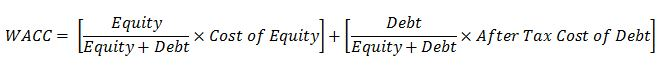## Weighted Average Cost Of Capital (WACC)Weighted Average Cost Of Capital (WACC) is a calculation of company cost of capital which is based on a weighted average between debt and equity. Its mathematical expression is listed below;WACC represents the minimum return on investment that can be a base line for a company to make a decision for new projects. Generally, companies will accept only projects that have returns more than WACC.

This example will use the previous information from Cost of Debt and Cost of Equity article to demonstrate WACC calculation.Continue reading